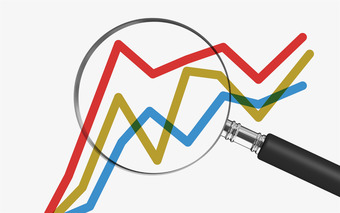# Statistics 101 | Online | Melbourne

The amount of data generated in the world continues to accelerate, and with it, the opportunity to analyse this data to manage businesses better, design better products, and much more.

But this raises many important questions, like how much data do we need to answer the questions we’ve posed? How do we know that the conclusions we have drawn are actually valid? How do we display these conclusions in a meaningful and useful way?

The answers to these questions are found in… statistics! Come and learn the basics of statistics, the science of collecting, summarising, and drawing valid conclusions from data.

Topics we will cover

• Overview of fundamental statistical concepts
• Calculating mean, median, mode, and standard deviation
• Creating charts such as box plots and scatter plots
• Understand correlations and apply regression analysis
• Conducting hypothesis testing with t-tests

### Takeaways

• Define the science of statistics and its use
• Understand fundamental statistical concepts
• Apply statistical analysis in Excel to draw valid conclusions